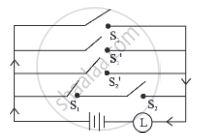# Construct the switching circuit of the following: p ∨ (∼ p) ∨ (∼ q) ∨ (p ∧ q) - Mathematics and Statistics

Sum

Construct the switching circuit of the following:

p ∨ (∼ p) ∨ (∼ q) ∨ (p ∧ q)

#### Solution

Let p: the switch S1 is closed
q: the switch S2 is closed
r: the switch S3 is closed
∼ p: the switch S1′ is closed or the switch S1 is open
∼ q: the switch S2′ is closed or the switch S2 is open
∼ r: the switch S3′ is closed or the switch S3 is open.

Then the switching circuit corresponding to the given statement pattern is:Concept: Application of Logic to Switching Circuits
Is there an error in this question or solution?